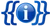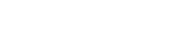Documentation
Namenumber / number
semi
some caption
damage
number
heat / cool
number
firerate
number
brst delay
number
deviation
number
recoil / ctrl
number
R/E time
number
perks
lets go here
```{{Weapon Flashcard
| name			= {{PAGENAME}}
| image			= Image.png
| class-name	=
| ammo			= number / number
| fire-mode		= semi
| caption		=
<!-- Value Block -->
| damage                = number
| heat          	= number
| firerate		= number
| burst	                = number
| deviation		= number
| recoil-ctrl	        = number / number
| re-time		= number / number
| modules		= <li></li>
}}
```

Weapon flashcards with stats etc.